# Vector-MKdV Solitons I

## Vector MKdV Equation ut + 24|u|2ux + uxxx = 0

In vector notation, u = (u1,...,uN) is a N-component variable satisfying the vector MKdV equation

ut + 24 uu ux + uxxx =0

For the special case when N=2, this vector equation is equivalent to the Hirota-MKdV equation
ut + 24 uuux + uxxx =0
in complex-variable notation u = u1 + i u2.

### 1-Soliton Solution

Travelling waves are given by the 1-soliton solution

u(t,x)= (G/F) φ
with
G= √c, F= 2cosh(√c ξ)
where c > 0 is the speed, φ is the orientation unit vector, and ξ = xct is a moving coordinate.

### 2-Soliton Solution

Collisions where a fast travelling wave with speed c1 and orientation (unit vector) φ1 overtakes a slow travelling wave with speed c2 and orientation (unit vector) φ2 are given by the 2-soliton solution

u(t,x)= (G1/F) φ1 + (G2/F) φ2
with
G1 =(c1c2) √c1 cosh(√c2 ξ2), G2 =(c1c2) √c2 cosh(√c1 ξ1)
F= 4√c1c2 φ1φ2 + (√c1 − √c2)2 cosh(√c1 ξ1 + √c2 ξ2) + (√c1 + √c2)2 cosh(√c1 ξ1 − √c2 ξ2)

where ξ1 = xc1t, ξ2 = xc2t are moving coordinates.

### Interaction Properties

Depending on their speed ratio c1/c2 and relative orientation angle Δφ = arccos(φ1φ2), the fast and slow waves in a collision exhibit three different types of nonlinear interactions.

1) Merge-Split: the waves first merge together at t=x=0 and then split apart into their original shapes and speeds.
2) Bounce-Exchange: the waves first bounce and then exchange their shapes and speeds at t=x=0.
3) Absorb-Emit: the fast wave gradually first absorbs and then emits the slow wave.

In all three types of interactions, the waves emerging from the collision retain the shape, speed, and orientation of the initial two waves. The only remnant of the interaction is a forward shift in the position of the faster wave

Δx1 = (2/√c1) ln ( (√c1 + √c2)/(√c1 − √c2) ) > 0

and a backward shift in the position of the slower wave

Δx2 = (2/√c2) ln ( (√c1 − √c2)/(√c1 + √c2) ) < 0

where these position shifts depend only on the speeds of the waves but not on their orientations.

### Overlay of 1-Soliton Solutions and Corresponding 2-Soliton Solution

#### Merge-Split Interactionspeed ratio: c1/c2 = 2.8
relative orientation angle: Δφ = 0.75 π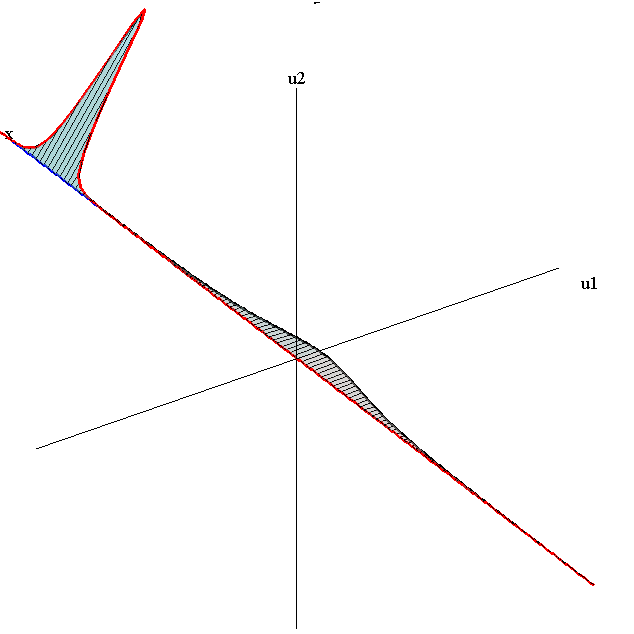speed ratio: c1/c2 = 50
relative orientation angle: Δφ = 0.3 π

#### Bounce-Exchange Interaction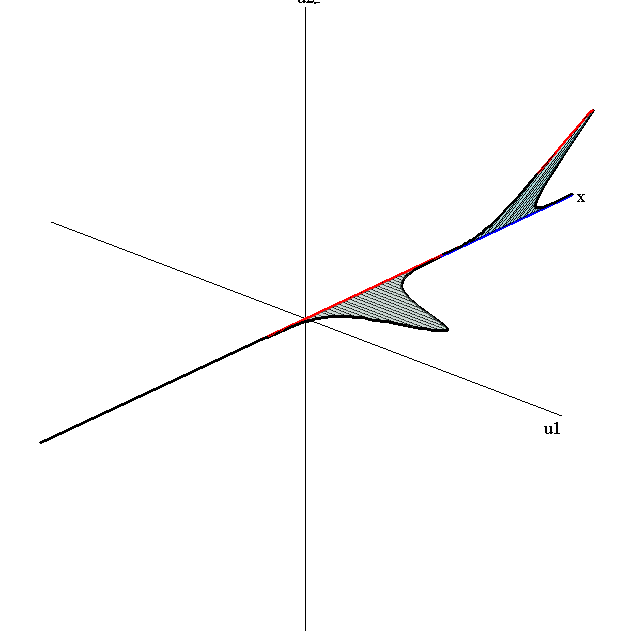speed ratio: c1/c2 = 2.8
relative orientation angle: Δφ = 0.3 π

#### Absorb-Emit interaction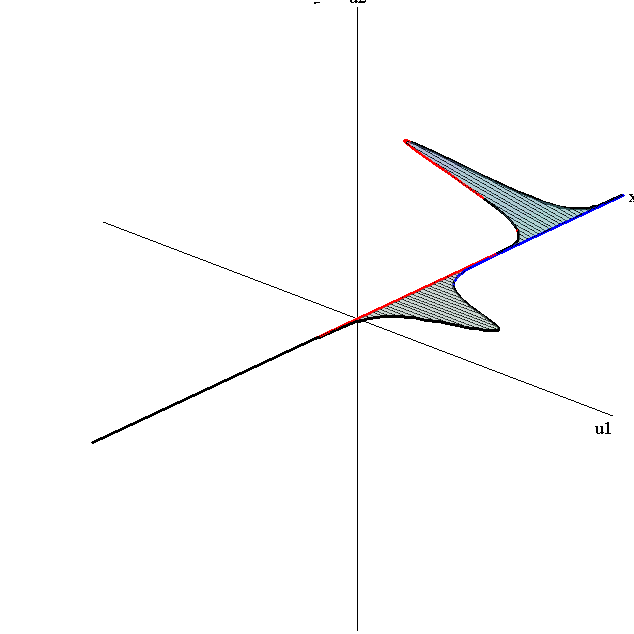speed ratio: c1/c2 = 2.8
relative orientation angle: Δφ = 0.95 π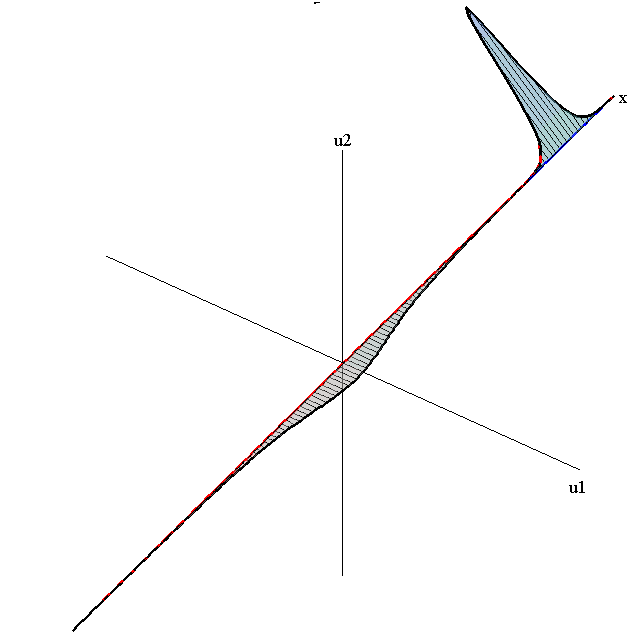speed ratio: c1/c2 = 50
relative orientation angle: Δφ = 0.75 π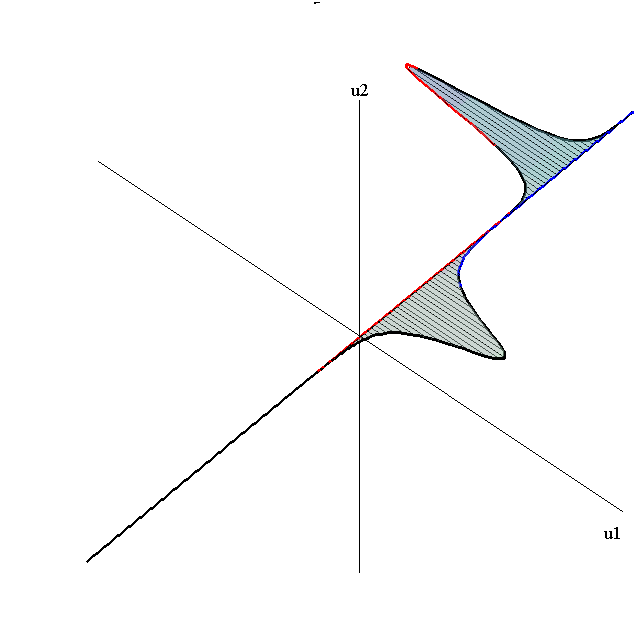speed ratio: c1/c2 = 9
relative orientation angle: Δφ = π